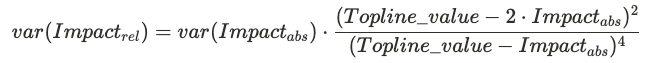# Topline and Projected Impact

The topline impact is the measured effect that an active experiment has on the overall topline metric each day. This is the real impact that results from running the experiment with its current allocation. The projected launch impact is an estimate of the topline impact we expect to see if a decision is made and the test group is launched to all users. This impact is computed relative to the expected baseline value of the topline metric if the experiment wasn't running at all.

## Computing Topline Impact​

The topline impact is computed daily and averaged over the selected dates. The exact calculation depends on whether the metric represents an absolute quantity or a ratio.

### Count and Sum Metrics (event_count, event_dau, sum)​

The absolute topline impact is derived directly from the experiment results. It depends on the difference in means between test and control, and the number of users in the test group.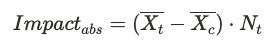Knowing the absolute impact and the overall metric value (as seen in the metrics dashboard), we can compute the relative impact. This is the percentage change in the overall daily metric value that is attributed to the active experiment.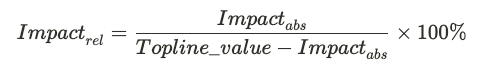### Ratio and Mean Metrics​

To properly derive the topline impact on a ratio metric we must understand the impact on the numerator (X) and denominator (Y) separately. The topline impact is the current value of the ratio metric minus the baseline value we obtain by subtracting the numerator and denominator impacts: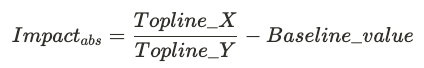Where the baseline value is the expected value of the topline metric if the experiment wasn't running: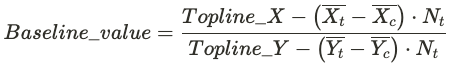The relative impact for ratio metrics is obtained by dividing the absolute impact by the baseline value: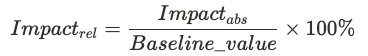## Computing Projected Launch Impact​

The layer allocation of the experiment and the size of the test group are used to estimate a scaling factor m, which represents the increase in absolute impact expected when a decision is made to launch the test group.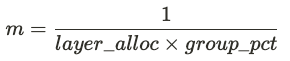Note that the targeting gate isn't factored in. The projected impact calculation assumes that the target gate remains the same after the experiment is launched.

### Count and Sum Metrics (event_count, event_dau, sum)​

For count and sum metrics, the projected absolute impact is simply the current topline impact scaled by a factor of m. For example: Consider an experiment running with 50% layers allocation and 50/50 test/control split, so that 25% of all users are in the test group. If the topline impact is currently +10 events per day, then launching the experiment would lead to +40 events per day.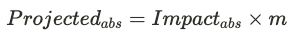The relative projected impact is expected percentage change in the topline metric, relative to the baseline value of the metric without the experiment running.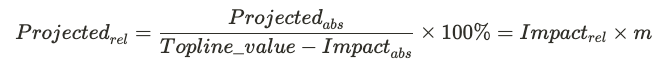### Ratio and Mean Metrics​

Similar to the topline impact calculation above, the projected impact of ratio metrics depends on the numerator and denominator impacts. We use the same scaling factor m to obtain the projected impact for each term:Where the first term represents the projected metric value after launch.

Finally, the projected relative impact of a ratio metric is the projected absolute impact divided by the baseline value of the ratio:## Confidence intervals​

The confidence intervals for topline and projected impact are computed in the same way as the confidence intervals for experiment deltas.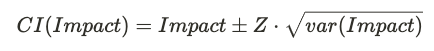In the case of absolute impact of count and sum metrics, the variance calculation is simply a linear combination of the test and control variances: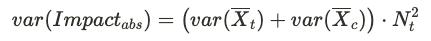And for projected launch impact we get: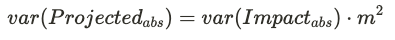For ratio metrics and relative impacts, the variance is calculated using the Delta method. This properly accounts for the correlation between the various numerator and denominator terms, leveraging Taylor expansion to linearize expressions containing non-linear combinations of experiment variables.

For example, the variance in the relative impact of a count metric is given by: